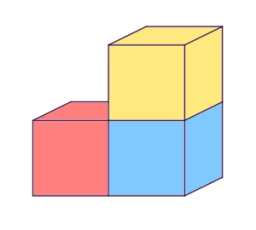# Calculate 81553

The picture puzzle made of wooden cubes weighs 0.792 kg. One cube has an edge length of 4 cm and a weight of 33 grams. Find out how many cubes make up a puzzle and calculate the wood density in kg/m³.

n =  24
ρ =  515.625 kg/m3

### Step-by-step explanation:Did you find an error or inaccuracy? Feel free to write us. Thank you!

Tips for related online calculators
Do you know the volume and unit volume, and want to convert volume units?